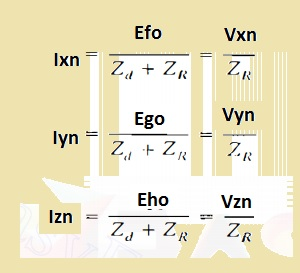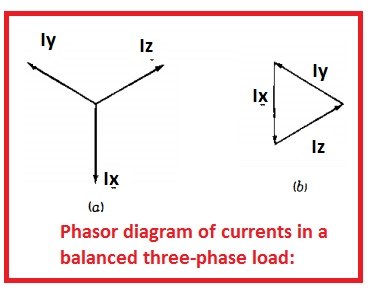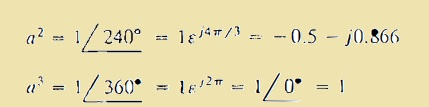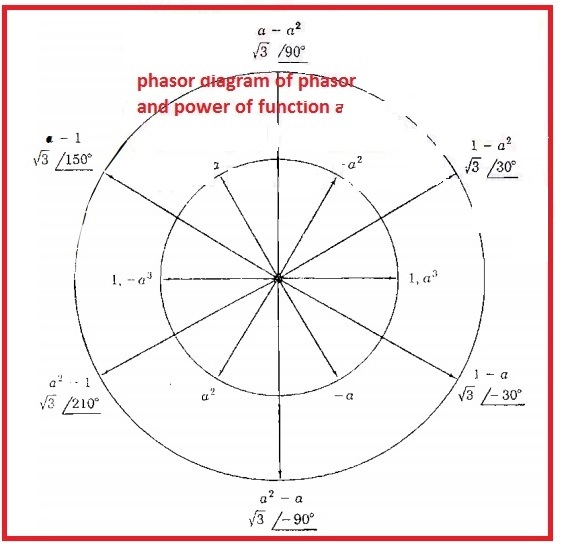Hello, friends welcome to the new post. In this post, we will have a detailed look at Balanced Three-Phase Circuits Voltage and Current Calculation.  the system having the same magnitude of three phases but with a difference of one twenty-degree of phase, the angle is called a balanced three-phase system. Here we are going to discuss the details about the voltage and current flowing in this system.

Generally, a three-phase system is used for the transmission of volts from one point to other from generation to load end. So let get started

## Balanced Three-Phase Circuits Voltage and Current Calculation

• In a power system, the power is generated through the use of three-phase generators.
• The load linked to the three phases of generators is balanced it means the impedance values are the same.
• in the below figure the generator configured with wye connection having natural wire n.• To understanding of this circuitry let us assume that the value of impedance and load is zero and also between points 0 and n also zero
• the resultant circuitry of this generator has emf in very phase and denoted with a circle.
• Each emf is configured in a series combination to the resistance and inductive reactance linked to Zd.
• The points x y and z are reference points
• and terminals of generated with f g h
• In this circuitry, the value of emf denoted with Exo, Eyo, and Ezo has equal magnitude and separated with one twenty degree of angle
• Let suppose that value of every emf is one hundred volts then Eao was taken as a reference then we have
• Exo = 100∠0V
• Eyo = 100∠240V
• Ezo = 100∠120V
• Here phase equation is fgh.
• In other words Exo leads  Eyo by one twenty-degree and Eyo leads by  Ezo one twenty degrees.
• In below figure, we can see the phase sequence• The voltage about the terminal is given here.
• Vfo=Exo-IfnZd
• Vgo=Eyo-IgnZd
• Vho=Ezo-IhnZd
• as o and n has the same value of potential so Vfo Vgo Vho has the same value of Vxn, Vyn, Vzn
• Equation of line current are mention here• Exo, Eyo Ezo has the same value of and one twenty-degree phase difference.
• Vfo, Vgo Vho also has hs same values and one twenty degrees phase difference
• In below figure you can see the 3 currents flowing in the system having balance configuration. In figure denoted as b their closed triangle is shown.• So the curent flowing in the neutral is zero.
• in case of nonbalance load, the current summation is zero and the current is passing among the points n and o.
• in the non-balance system, there is not the same potential till they are linked to 0 value of impedance.
• Due to phase variation in the voltage and current in case of a balanced three-phase system, it is easy to simple to use such a technique that denoting th phasor through one twenty degrees.
• The outcome of the product of 2 complicated numbers is the multiple of their magnitude and angle sum
• If the complicated number denoting phasor is multiplied to a complex number of unity magnitude and angle θ the resultant complex number denotes phasor equal to real phasor parted with the angle of θ.
• The complex number if unity value and angle is a function which that moves the phasor at which it works with angle
• In math the operator, j caused the rotation of ninety degrees and operator -1 that results in one eight degrees.
• 2 continues usage of operator J results in 90+90 that results in j x j cause to rotation at an angle off one-eighty degree.
• The a alphabet is generally used to represent the function that results in the movement of one twenty degrees anti-clock wises.
• This function is a complex number having a unity value having an angle of one twenty-degree and works as• If we use function a two time then phasor will be moved with the angle of two forty degrees.
• With using three times angle move to three-sixty degrees• In below diagram, we can see the phasor denotation having different values of a.• The line to lin voltage for below circuitry are Vfg, Vgh and Vhf• Following the path from f to g to the point n we have
• Vfg=Vfn+Vgn=Vfn-Vgn
• as the Exo and Vfn has not the same phase so we are replacing Vxn in place of Exo
• So in the below diagram shows the phasor diagram of voltage to neutral and vlaeu of Vfg is given here.

• In case of function we have

Vgn=a2Vgn

Vfg=Vfn-a2Vfn

• phasor equation
• In the case of phasor Vfg leading the Vfn through an angle of thirty degrees and has √3  has a large value.
• Other value of line to line can be found through using this method  In the above figure we can all values of line to line volts
• the value of balance their phase line to line volts of three-phase circuitry same to √3 time the magnitude of line to neutral

So that is all about the Balanced Three-Phase Circuits Voltage and Current Calculation. If you have any further query ask in the comments box. See you in the next post